By Andy May

Generally, it is agreed that the Earth’s top-of-atmosphere (TOA) energy budget balances to within the margin of error of the estimates (see Kiehl and Trenberth, 1997). The incoming energy, after subtracting reflected energy, is thought to be roughly 239 W/m2 which matches, within the margin of error, the outgoing energy of roughly 239 W/m2. Satellite data suggest TOA energy imbalances of up to 6.4 W/m2 (Trenberth, et al., 2008). However, Zhang, et al. (2004) suggest that the uncertainty in the TOA measurements is 5-10 W/m2 and the uncertainty in the surface radiation absorption and emission is larger, 10-15 W/m2. We examine some potential causes for these uncertainties.

To compute the magnitude of the greenhouse effect, the TOA incoming and outgoing radiation is usually compared to the Earth’s radiation emissions due to its overall average surface temperature of approximately 288K (14° to 15°C) according to the HADCRU version 4 1961-1990 baseline absolute temperature dataset. Using Planck’s function or the similar Stefan-Boltzmann law (see figure 1), the radiation emitted by the Earth can be calculated from its temperature (T), if we assume the Earth acts like a blackbody. Normally the radiation calculation is done assuming an emissivity (e) of 1, which means the Earth is a perfect blackbody that emits as much energy as it receives. The area used is one square meter, so the result is given in Watts/m2. Using these assumptions, the calculation results in the Earth emitting about 390 W/m2 (Kiehl and Trenberth, 1997) for a surface temperature of 288K.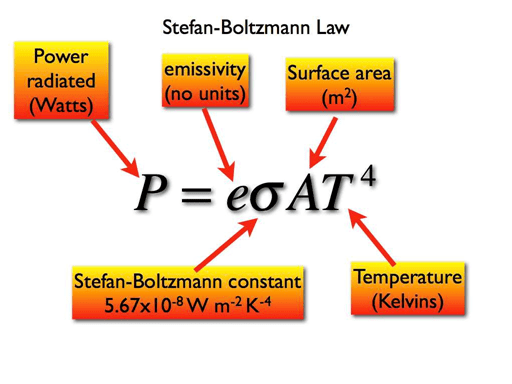Figure 1 and Equation 1 (source)

The greenhouse effect (GHE), when calculated this way, shows an imbalance of 390-239=151 W/m2. Kiehl and Trenberth, 1997 calculated a similar overall forcing of 155 W/m2 using the same procedure. This GHE calculation makes a lot of assumptions, not the least of which is assuming the Earth has an emissivity of 1 and is a blackbody. But, here we want to consider the problem of using a global average temperature (T) for the Earth, which is a rotating sphere, with only one-half of the sphere facing the Sun at any one time.

One specific problem is that the Earth is not at a uniform global temperature. If it averages 288K, then there will be places on the planet that are 288K and those spots will emit roughly 390 W/m2. But, much of the planet will be at a different temperature and will emit energy proportional to T4. The average of T taken to the fourth power is not the same as the average of T4. This is clear from basic high school algebra, so how much difference does this make?

To answer that we will turn to the Hadley Climate Research Unit (HADCRU) version 4 global temperature database. We will use their version 4 baseline 1961-1990 absolute temperature dataset and their 1850 to 2017 temperature anomaly dataset. The construction of the baseline and the anomaly datasets is described in Jones, et al. (2012). Since the temperature series anomalies are anomalies from each series’ 1961-1990 average, we should be able to use the series baseline temperature to convert the anomalies to actual temperatures. These are both 5° x 5° gridded datasets. Anomalies are computed for each station to avoid problems with elevation differences, etc. This is done before they are gridded. Thus, adding the baseline temperature to the anomaly does not restore the original measurements. To quote from the HADCRU web site:

“Stations on land are at different elevations, and different countries measure average monthly temperatures using different methods and formulae. To avoid biases that could result from these problems, monthly average temperatures are reduced to anomalies from the period with best coverage (1961-90). For stations to be used, an estimate of the base period average must be calculated. Because many stations do not have complete records for the 1961-90 period several methods have been developed to estimate 1961-90 averages from neighbouring records or using other sources of data (see more discussion on this and related points in Jones et al., 2012). Over the oceans, where observations are generally made from mobile platforms, it is impossible to assemble long series of actual temperatures for fixed points. However, it is possible to interpolate historical data to create spatially complete reference climatologies (averages for 1961-90) so that individual observations can be compared with a local normal for the given day of the year (more discussion in Kennedy et al., 2011).

It is possible to obtain an absolute temperature series for any area selected, using data from the absolute file, and then add this to a regional average of anomalies calculated from the gridded data. If for example a regional average is required, users should calculate a regional average time series in anomalies, then average the absolute file for the same region, and lastly add the average derived to each of the values in the time series. Do NOT add the absolute values to every grid box in each monthly field and then calculate large-scale averages.”

By the way, “NOT” is capitalized on the website, I did not change this. My plan was to add the grid 1961-1990 temperature to the grid anomaly and get an approximate actual temperature, but they say, “do NOT” do this. Why tell the reader he can add the absolute 1961-1990 baseline temperature average to averaged anomalies and then expressly tell him to not add the absolute temperature grid to an anomaly grid? Every anomaly series must be referenced to its own 1961-1990 average, why does it matter if we average the anomalies and the absolute baseline temperatures separately before adding? So, naturally, the first thing I did was add the absolute 1961-1990 grid to the anomaly grid for the entire Earth from 1880 to 2016, precisely what I was instructed “NOT” to do. The absolute temperature grid is fully populated and has no missing values. The year-by-year anomaly grids have many missing values and the same cells are not populated in all years, it turns out this is the problem that HADCRU are pointing to in this quote.

Figure 1 shows the 1880 to 2016 global average temperatures computed the way HADCRU recommends. I first averaged the anomalies for each year, weighted by the cosine of latitude because it is a 5° x 5° grid and the area of each grid cell decreases from the equator to the poles with the cosine of the latitude. Then I add the global average 1961-1990 temperature to the average anomaly. While the baseline temperature grid is fully populated with absolute temperatures, the yearly anomaly grids are not. Further the populated grid cells come and go from year to year.  This process mixes a calculation from a fully populated grid with a calculation from a sparsely populated grid.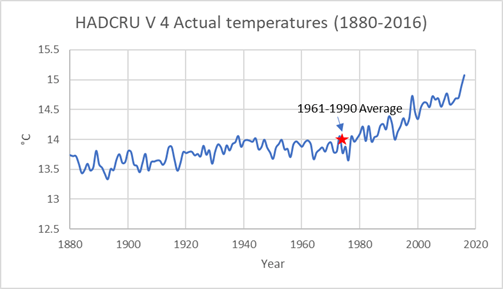Figure 1, Average the anomalies and then add the average 1961-1990 global temperature

By doing it in the way they expressly advise us not to do, we obtain figure 2. In figure 2, I add the appropriate 1961-1990 absolute cell average temperature to each populated grid cell, in each year, to create a grid of absolute temperatures and then average that, ignoring null cells.  In this process, the absolute temperature grid matches the anomaly grid.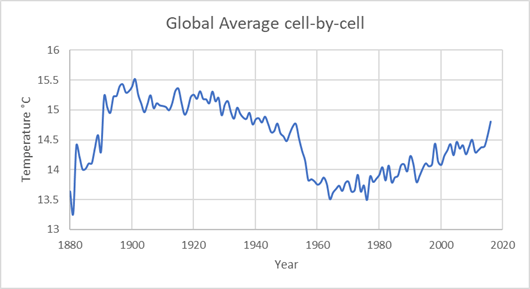Figure 2, Convert each grid cell to actual temperature, then average

The difference between figures 1 and 2 is most apparent prior to 1950. After 1950, the lower plot is a few tenths of a degree lower, but the trend is the same. With perfect data, the two plots should be the same. Each time series is converted to an anomaly using its own 1961-1990 data, multiple series in each grid cell are merged using straight averages. But, the data are not perfect. Grid cells are populated in some years and not in other years. Prior to 1950, northern hemisphere coverage never exceeds 40% and southern hemisphere coverage never exceeds 20%. Given the wide discrepancy between figures 1 and 2, it is not clear the data prior to 1950 is robust. Or, stated more clearly, the data prior to 1950 is not robust. It is also not clear why the period 1950 to 2016 is 0.2 to 0.3°C cooler in figure 2 than in figure 1, I’m still scratching my head over that one.

The HADCRU procedure for computing global temperatures

The procedure for computing the HADCRU version 4 grid cell temperatures is described on their web site as follows:

“This means that there are 100 realizations of each [grid cell] in order to sample the possible assumptions involved in the structure of the various components of the error (see discussion in Morice et al., 2012). All 100 realizations are available at the above Hadley Centre site, but we have selected here the ensemble median. For the gridded data, this is the ensemble median calculated separately for each grid box for each time step from the 100 members. For the hemispheric and global averages, this is again the median of the 100 realizations. The median of the gridded series will not produce the median of the hemispheric and global averages, but the differences will be small.”

Thus, the HADCRU version 4 global average temperature is not a true average. Instead it is the median value of 100 statistical realizations for each populated grid cell and both hemispheres. Every temperature measurement contains error and is uncertain. The 5° x 5° latitude and longitude grid created by HADCRU contains 31,104 grid cells. Most of these have no value, figure 3 shows the number of these cells that are null (have no value) by year from 1880 through 2016.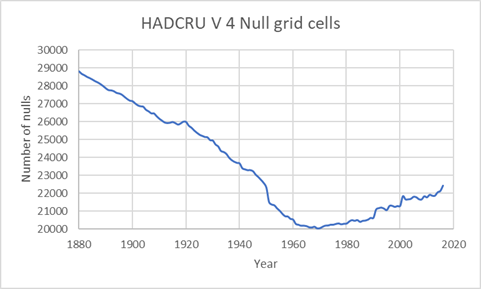Figure 3: (Data source)

As you can see, most of the cells have no data, even in recent years. In figure 4 we can see the distribution of populated grid cells. The cells with adequate data are colored, those with insufficient data are left white. Coverage of the northern hemisphere approaches 50% from 1960-1990, coverage of the southern hemisphere never exceeds 25%.Figure 4 (source: Jones, et al., 2012)

So, the data is sparse and most of the data is on land and in the northern hemisphere. Both poles have little data. So HADCRU has two problems, first how to deal with measurement uncertainty and second how to deal with the sparse and uneven distribution of the data. Measurement uncertainty is dealt with by requiring that each grid cell have a sufficient number of stations reporting over the year being considered. Since the baseline period for the temperature anomalies is 1961-1990, sufficient measurements over this period are required also. Generally, they require the stations to have at least 14 years of data between 1961 and 1990. Stations that fall outside five standard deviations of the grid mean are excluded.

The monthly grids are not contoured to fill in the missing grid values as one might expect. Once the median temperature is computed for each grid cell with sufficient data, the populated grid cells are cosine-weighted and averaged, see equation 9 in Morice, et al., 2012. The area varies as the cosine of the latitude, so this is used to weight the grids. The weighted grid values are summed for each hemisphere, averaging the hemispheres results in a global average temperature. Seasonal and yearly averages are derived from monthly grid values.

Most of the populated grid cells are on land because this is where we live, yet 71% of the surface of the Earth is covered by ocean. Currently, this is not a problem because we have satellite estimates of the sea-surface temperature and the atmosphere above the oceans. In addition, we have the ARGO buoy network that provides high quality ocean temperatures. Yet, historically it has been a problem because all measurements had to be taken from ships. The critical HADSST3 dataset used to estimate ocean temperatures is described by Morice, et al., 2012. A fuller explanation of the problems estimating ocean grid cell historical temperatures is found in Farmer, et al., 1989. The data used prior to 1979, are from ship engine intakes, drifting buoys, and bucket samples taken over the sides of ships. These sources are mobile and prone to error. The ocean mixed layer is, on average, 59 meters thick (JAMSTEC MILA GPV data). See more on the JAMSTEC ocean temperature data here. The mixed layer is the portion of the ocean that is mostly in equilibrium with the atmosphere. This layer has 22.7 times the heat capacity of the entire atmosphere and exerts considerable influence on atmospheric temperatures. It is also influenced by the cooler, deeper ocean waters and can influence them due to ocean upwelling and downwelling (see Wim Rost’s post here).

My calculations

I started with the 1961-1990 baseline temperature data, called “Absolute” and found here. This is a series of monthly 5°x5° global temperature grids for the base period. Unlike the anomaly datasets, these grids are fully populated and contain no null values, how the Absolute dataset was populated is explained in Jones, et al., 2012. Figure 5 is a map of the average Absolute temperature grid.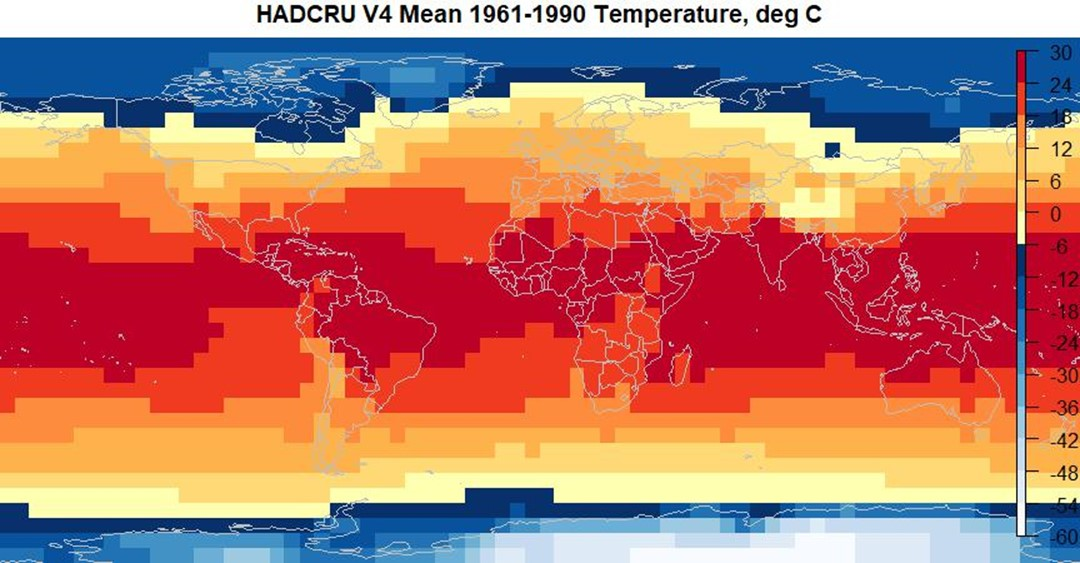Figure 5, Map of the “Absolute” data (data source: HADCRU)

My procedure is like the one used by HADCRU. I first read the Absolute grid, it populates an array dimensioned by 72 longitude 5° segments, 36 5° latitude segments, and 12 months or one year. Next, I break the HADCRUT4 global anomaly grid down year-by-year, average the populated cells, and then add the average Absolute 1961-1990 temperature to the average anomaly. The results are shown in figure 1. As discussed above, I also spent some time doing exactly what the HADCRU web site says I should “NOT” do, this result is shown in figure 2.

The HADCRU data go back to 1850, but there is very little global data before 1880 and much of it was taken in the open air. Louvered screens to protect the thermometers from direct sunlight were not in wide use until 1880, this adversely affects the quality of the early data. So, I only utilize the data from 1880 through 2016.

The surprising thing about the graph in figure 2 is that the temperatures from 1890 to 1950 are higher than any temperatures since then. Refer to figure 3 for the number of null values. There are 31,104 cells total, the maximum number that are populated is around 11,029 in 1969 or 35%. Figure 6, inverts figure 3 and shows the number of populated cells for each year.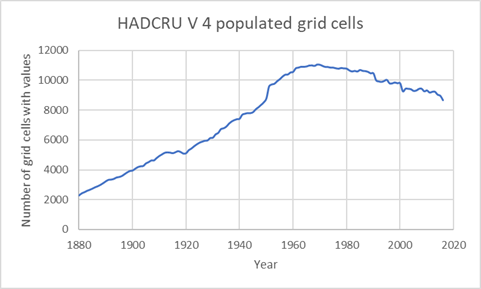Figure 6

Is the higher temperature from 1890 to 1950 in figure 2, due to the small number of populated cells? Is it due to the uneven distribution of populated cells? There is a sudden jump in the number of populated grid cells about 1950 that coincides with an anomalous temperature drop, what causes this? Is it due to an error I made in my calculations? If I did make an error (always possible) I have every confidence someone will find it and let me know. I’ve been over and over my R code and I think I did it correctly. I’ve read the appropriate papers and can find no explanation for these anomalies. All the data and the R code can be downloaded here. Experienced R users will have no problems, the zip file contains the code, all input datasets and a spreadsheet summary of the output.

Power and Temperature

The original reason for this study was to see what difference the computational sequence makes in computing the energy emissions from the Earth. That is, do we take the fourth power of an average temperature as done by Kiehl and Trenberth, 1997? Or, do we take each grid cell temperature to the fourth power and then average the Stefan-Boltzmann (SB) power from equation 1? The average of the 2016 HADCRU temperatures is 15.1°C. The SB energy emissions computed from this temperature (288K) are 391 W/m2 as commonly seen in the literature. If we compute the SB emissions from all the populated HADCRU grid cells in 2016 and average them, weighted by area, we get 379 W/m2. This is a small difference unless we compare it to the estimated difference that increasing CO2 might have. In the IPCC AR5 report, figure SPM.5 (page 14 of the report or you can see it here in the third figure) suggests that the total effect of man’s CO2 emissions since 1750 has been 2.29 W/m2, much less than the difference between the two calculations of the Earth’s emissions.

The comparison gets worse when we look at it over time. Figure 7 shows the computation of power emissions computed using a global average temperature or (Mean T)4. Figure 8 shows the calculation as done on each populated grid cell and then averaged or (Mean T4).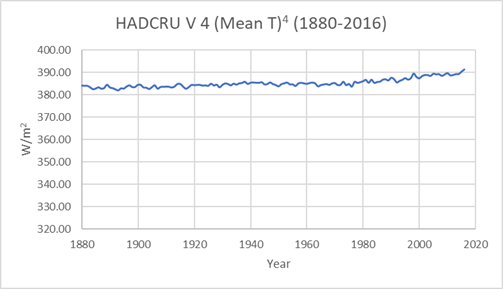Figure 7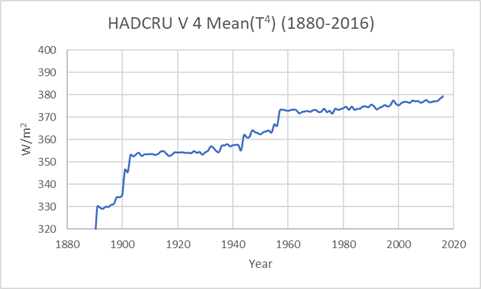Figure 8

It seems likely that the differences from 1880 to 1950 are related to the number of populated cells and their distribution, but this is speculation at this point. One must wonder about the accuracy of this data. The comparison since 1950 is OK, except for the algebraic difference due to averaging temperature first or taking each temperature to the fourth power first and then averaging power. From 1950 to 2014, this difference averages 13 W/m2.

Discussion and Conclusions

I do not challenge the choice HADCRU made when they decided to create 100 statistical realizations of each grid cell and then choose the overall median value, weighted by cosine(latitude), as the average temperature for each hemisphere and then combine the hemispheres. This is a reasonable approach, but why is the result so different from a straightforward weighted average of the populated grid cells? To me, any complicated statistical output should line up with the simple statistical output, or the difference needs to be explained. The comparison between the two techniques over the period 1950 to 2016 is OK, although the HADCRU method results in a suspiciously higher temperature. I suspect the data from 1950 to 2016 is much more robust than the prior data. I would doubt any conclusions dependent upon the earlier data.

Their recommended calculation process is a bit troubling.  They recommend averaging a sparse anomaly grid, then averaging a completely populated absolute temperature grid, and then sum the two averages.  Then they explicitly instruct us not to select the same population of grid cells (anomaly and absolute), sum those, and then average.  Yet, the latter technique sums apples to apples.

Finally, it is very clear that using the SB equation to compute the Earth’s energy emissions with an estimated global average temperature is incorrect, this is how the emissions were computed in figure 7. When we compute the SB emissions from each HADCRU populated grid cell and then average the result, which basic algebra tells us is the correct way, we get the result in figure 8. Comparing the two suggests that there are significant problems with the data prior to 1950. Is this the number of null grid cells? Is it the areal distribution of populated grid cells? Is it a problem with estimated sea surface temperatures? Or perhaps some other set of problems? Hard to say, but it is difficult to have much confidence in the earlier data.

We are attempting to determine the effect of an increase in CO2. This results in an estimated “forcing” of about two W/m2. We also want to know if temperatures have increased one-degree C in the last 140 years. Is this data accurate enough to even resolve these effects? It is not clear to me that it is.

The R code and the data used to make the figures in this post can be downloaded here.